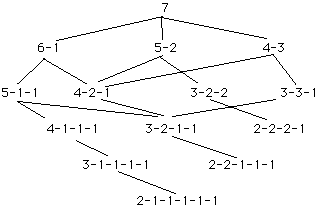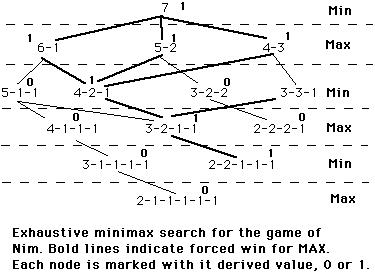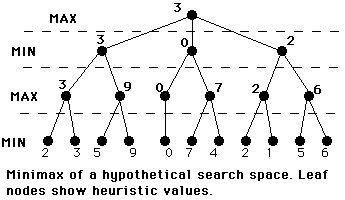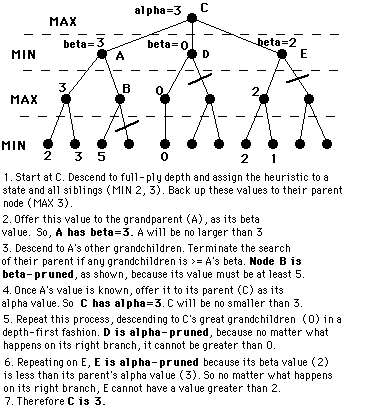## Using Heuristics in Games

Games are an important test-bed for heuristic algorithms. Two-person games are more complicated than a simple puzzle because they involve an unpredictable opponent.

### Minimax Procedure

The Game of Nim: A number of tokens are placed on a table between two opponents. At each move the player must divide the a pile of tokens into two nonempty piles of different sizes. Thus, 6 tokens may be divided into 5 and 1, 4 and 2, but not 3 and 3. The first player who is unable to make a move loses the game.

For a small number of tokens the search space can be searched exhaustively. The next figure gives the complete space for a 7-token game.In a two-person game, you must assume that your opponent has the same knowledge that you do and applies it as well as you do. So at each stage of the game you must assume your opponent makes the best available move. This is the basis of the minimax procedure.

In minimax, the players are referred to as MAX (the player) and MIN (the opponent). Both try to maximize their moves. MAX is the player, trying to MAXimize her score. And MIN is the opponent trying to MINimize MAX's score.

#### Minimax Procedure on a Complete Search Space

1. Label each level of the search space according to whose move it is at that level.

2. Starting at the leaf nodes, label each leaf node with a 1 or 0 depending on whether it is a win for MAX (1) or MIN (0).

3. Propagate upwards: if the parent state is MAX, give it the MAX of its children.

4. Propagate upwards: if the parent state is MIN, give it the MIN of its children.

Consider the minimax graph for the game of Nim. The value at each state represents the value of the best state that this player can hope to achieve. The derived values are used to choose among alternative moves.You should be able to answer the following questions by analyzing this graph.

• Who makes the first move in this particular game?
• Under what circumstances will MIN win the game?
• Suppose MAX is in the middle state (5-2) on her first move. What move does minimax recommend.
• How good are MAX's prospects in this game?

### Heuristic Minimax

For most games it is impossible to expand the graph to its leaf nodes. Instead an n-move look-ahead strategy is used. The state space is expanded to n levels. Each leaf node in this subgraph is given a value according to the heuristic evaluation function. Values then are propagated back to the root node. The value propagated back represents the heuristic value of the best state that can be reached from that node.Example: Samuel's checkers program used a weighted sum as the evaluation function. It used a simple learning algorithm to adjust the weights after wins and losses, so the program improved over time.

### The Alpha-Beta Procedure

Alpha-beta pruning is a procedure to reduce the amount of computation and searching during minimax. Minimax is a two-pass search, one pass is used to assign heuristic values to the nodes at the ply depth and the second is used to propagate the values up the tree.

Alpha-beta search proceeds in a depth-first fashion. An alpha value is an initial or temporary value associated with a MAX node. Because MAX nodes are given the maximum value among their children, an alpha value can never decrease; it can only go up. A beta value is an initial or temporary value associated with a MIN node. Because MIN nodes are given the minimum value among their children, a beta value can never increase; it can only go down.

For example, suppose a MAX node's alpha = 6. Then the search needn't consider any branches emanating from a MIN descendant that has a beta value that is less-than-or-equal to 6. So if you know that a MAX node has an alpha of 6, and you know that one of its MIN descendants has a beta that is less than or equal to 6, you needn't search any further below that MIN node. This is called alpha pruning.

The reason is that no matter what happens below that MIN node, it cannot take on a value that is greater than 6. So its value cannot be propagated up to its MAX (alpha) parent.

Similarly, if a MIN node's beta value = 6, you needn't search any further below a descendant MAX that has acquired an alpha value of 6 or more. This is called beta pruning.

The reason again is that no matter what happens below that MAX node, it cannot take on a value that is less than 6. So its value cannot be propagated up to its MIN (beta) parent.

#### Rules for Alpha-beta Pruning

• Alpha Pruning: Search can be stopped below any MIN node having a beta value less than or equal to the alpha value of any of its MAX ancestors.

• Beta Pruning: Search can be stopped below any MAX node having a alpha value greater than or equal to the beta value of any of its MIN ancestors.
Example: A left-to-right alpha-beta prune of the hypothetical minimax search space: